# Order of Operations

“Order of operations” is a phrase that describes the order in which you apply mathematical operations, such as addition and multiplication, when evaluating an algebraic expression. On the GMAT, some questions will hand you an algebraic expression and ask you what it equals; the key to such a question will be using order of operations. Or, in many other questions, you will perform algebra by setting up and working through equations, and during this process you will have algebraic expressions to evaluate (“evaluate” meaning “perform all the operations there are to be performed”). In this latter type of case, also, you’ll need to use order of operations.

In the United States, the order of operations is often expressed with the mnemonic PEMDAS: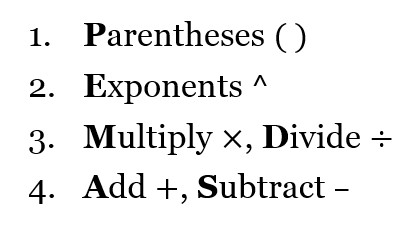The order of operations is Parentheses, Exponents, Multiplication and Division (from left to right), Addition and Subtraction (from left to right). This can be remembered as, “Please Excuse My Dear Aunt Sally.” Other variations of this mnemonic exist (such as BODMAS, outside the United States), but they all express the same rules.

To see the importance of order of operations, consider this example: evaluate the expression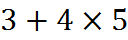If we were to add first, contrary to the rules, the expression gives us 7 times 5 and evaluates to 35. If we multiply first, as we should, the expression gives us 3 + 20 and evaluates to 23. The first evaluation is incorrect and the second evaluation is correct. The order of operations rule ensures that we always have one unique way to evaluate an expression.

If, in our example, we wanted to say that addition happened before multiplication in the example above, we would write it as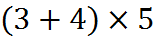and then the first way of evaluating it would be the correct way. Since parentheses come first in PEMDAS, they overrule the other rules and are used when the order of evaluation needs to be different from the EMDAS part of PEMDAS.

## Examples

Let’s evaluate these expressions.

1. a)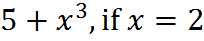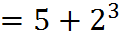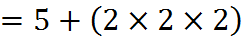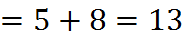b)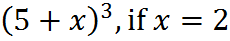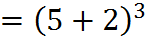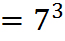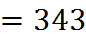2. a)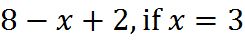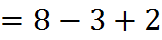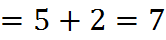b)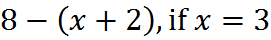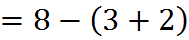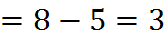Welcome! You are encouraged to register with the site and login (for free). When you register, you support the site and your question history is saved.